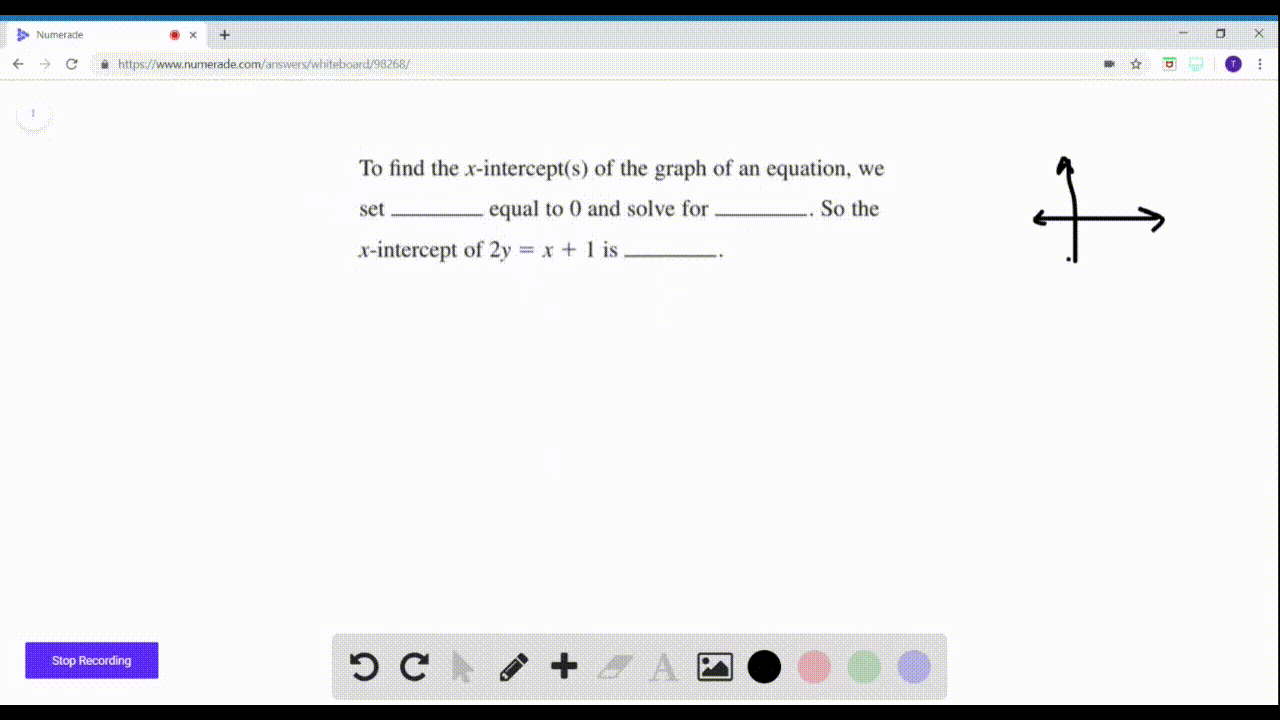🎉 The Study-to-Win Winning Ticket number has been announced! Go to your Tickets dashboard to see if you won! 🎉View Winning Ticket### To find the $x$ -intercept(s) of the graph of an …

01:16Problem 1

# If the point $(2,3)$ is on the graph of an equation in $x$ and $y$ then the equation is satisfied when we replace $x$ by _____ and $y$ by _____. Is the point $(2,3)$ on the graph of the equation $2 y=x+1 ?$ Complete the table, andsketch a graph.

## Discussion

You must be signed in to discuss.

## Video Transcript

this question asks a few questions within the question at birth, asks that if we were trying to determine if a 0.23 was on the equation of the line, the equation specifically in X and why, then the equation is satisfied when we're when we replace X bite, too. And why by three Here we're given the equation of the line to Why equals X Plus one. You were asked if the 0.2 threes on this line to answer that question. We was substitute in. The values is given. We put the two and for X the Y in for three. And when we evaluate here, we get that six equals three, which clearly is not true. So the ordered Pierre is not on the graph. Um were then asked to complete this table and sketch the graph. Um, this is going to be a little bit easier if we re work with this equation. I am going to substitute into why equals 1/2 X plus 1/2. So here, when we substituted negative too for X, you don't really have room to show that I could show it over here 1/2 times native to you know that? That gives us a negative one plus 1/2. That gives us negative 1/2. So the ordered Pierre is native to negative 1/2 when we substitute in and negative one. You see that we get zero when we substitute in zero, we will get 1/2. When we substitute in a one, we will get one and 1/2 and we substitute in a two. You will get two and 1/2 sono If we're putting this on the graph, we've got negative too negative. 1/2 negative one, is it? Zero zero is at 1/2 One is one and 1/2 too. Is that two and 1/2? I might have been a little bit off with that last point, but there we have. We have a sketch of the equation that was given